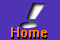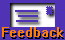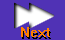the REXX Files - by Dr. Dirk TerrellEXX has some very powerful string-handling functions that can make the casual or professional programmer's life a lot easier. A common example of these is the string-handling function, PARSE. For those of you not up on the lingo, "parsing" means taking one string and splitting it into several others.

This function has several forms, and is extremely powerful. One variant of PARSE, PARSE ARG, is useful when you want to get the ARGuments that were passed to a program (or a function or subroutine).

Let's look at this function with a simple example. Suppose you need a REXX program to calculate the square of a number. You could write it like this:

```/* REXX program to compute the square of a number */
NUMBER=5
SQUARE=NUMBER*NUMBER
SAY "The square of" NUMBER "is" SQUARE
EXIT```
But you would quickly grow tired of having to edit the square.cmd file to change the NUMBER=... line every time you wanted to compute the square of a number. It would be better if you could run the program and tell it the number you wanted the square of at runtime. For example:
```C:\>square 5
The square of 5 is 25
C:\>```
In this case 5 is the "argument" of the program square.cmd. To make it work like this, we have to have some way of getting the 5 from the command line into the program. This is done with the PARSE ARG function like so:
```/* A more flexible program to compute squares of numbers */
PARSE ARG NUMBER
SQUARE=NUMBER*NUMBER
SAY "The square of" NUMBER "is" SQUARE
EXIT```
As a final note on PARSE ARG (and PARSE in general), you can split the command line into several parts (if they exist). Suppose you have a program that needs two numbers as input, for example a program that computes the density of a body. For density you need mass and volume, with density being mass divided by volume.
```/* Computes the density of a body given mass and volume as input */
PARSE ARG MASS VOLUME
DENSITY=MASS/VOLUME
Say "The density is" DENSITY
EXIT```
You can extend this behaviour to as many command line arguments as you like by adding more variables to the PARSE ARG line. If you include fewer variables after the PARSE statement than the user enters on the command line, the extra arguments are lumped together into the last variable listed after the PARSE statement. For example:
`parsetest.cmd one two three four`
with a PARSE ARG line in the .cmd file like:
`PARSE ARG a1 a2`
will set a1='one' and a2='two three four'

You can force extra command line arguments to be dropped if you add a . (period) at the end of the PARSE ARG line. For example:

`parsetest.cmd one two three four`
with a PARSE line like:
`PARSE ARG a1 a2 .`
will set a1='one' and a2='two' but ignore the 'three four'. That's it for now. Until next time...
Dr. Dirk Terrell is an astronomer at the University of Florida specializing in interacting binary stars. His hobbies include cave diving, martial arts, painting and writing OS/2 software such as HTML Wizard.--[Our Sponsor: Post Road Mailer - A high performance, 32-bit, email program.]